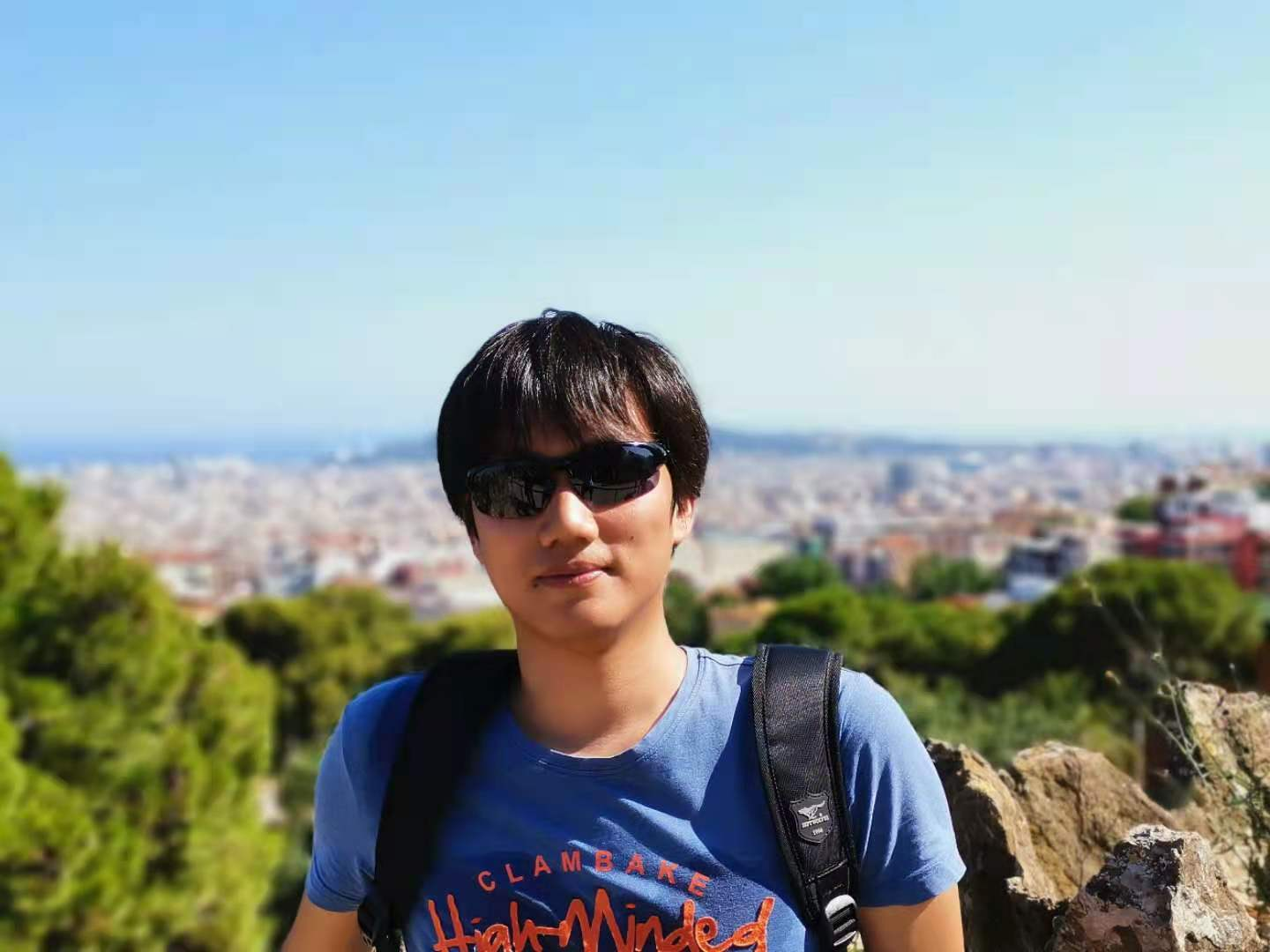# For: Evolution Strategies as a Scalable Alternative to Reinforcement Learning

542

## 方法

### 进化策略

\begin{align*} \nabla_{\psi} \mathbb{E}_{\theta \sim p_\psi} F(\theta) &= \nabla_\psi \int p_\psi(\theta)F(\theta) d \theta \\ &=\int F(\theta) \nabla_\psi p_\psi(\theta) d\theta\\ &=\int F(\theta) \cdot p_\psi(\theta) \nabla_\psi \log p_\psi(\theta) d \theta \\ &= \mathbb{E}_{\theta \sim p_\psi}\left[ F(\theta) \nabla_\psi \log p_\psi(\theta) \right] \tag{1} \end{align*}

$$\mathbb{E}_{\theta \sim p_\psi} F(\theta) = \mathbb{E}_{\epsilon \sim N(0, I)} F(\mu + \sigma \epsilon)$$

$$\nabla_\mu \mathbb{E}_{\epsilon \sim N(0, I)} F(\mu + \sigma \epsilon) = \mathbb{E}_{\epsilon \sim N(0, I)}\left [ F(\mu + \sigma \epsilon) \nabla_\mu \log \phi_{\mu, \sigma}(\mu + \sigma \epsilon) \right] ; 使用公式(1)$$

$$\nabla_\mu \log \phi_{\mu,\sigma}(\mu + \sigma \epsilon) = \cfrac{\epsilon}{\sigma}$$

$$\nabla_\mu \mathbb{E}_{\epsilon \sim N(0, I)} F(\mu + \sigma \epsilon) = \cfrac{1}{\sigma} \mathbb{E}_{\epsilon \sim N(0, I)} \left[ F(\mu + \sigma \epsilon) \epsilon \right]$$

\begin{align*} \mathbb{E}_{\epsilon \sim N(0, I)} \left[ F(\mu + \sigma \epsilon) \epsilon \right] \approx \cfrac{1}{n} \sum_{i=1}^{n}\left[ F_i \cdot \epsilon_i \right] \ \ \tag{2} \end{align*}

### 算法

4. 在参数更新前，对奖励值进行数量级的适配以让训练过程更加稳定
5. 对参数采用weight decay以防参数相对于扰动值$\epsilon$来说变得过大
6. 从算法中可以看出，每次运行都要完整运行完成一轮与环境的交互。而我们知道，与RL的环境交互时，有的策略运行步数长，有的则很短，这就有可能导致步数短的服务器CPU利用率不足。此时可以设置一个阈值$m$来限制最长运行步数。这个值可以动态调整（比如设置成所有进程平均步数的两倍）
7. 在Atari环境中，此方法出现了探索不足的问题，文中指出使用virtual batch normalization可以解决此问题，此方法与batch normalization非常相似，感兴趣可参考相关论文

### 优点

1. 无需计算梯度，在损失函数不可导情况下也可使用，并且无需担心long horizon问题
2. 仅需知道最终的奖励总和，因此无需解决RL中的奖励如何分配给每个步骤的问题，也无需担心不同环境中每一秒帧数不一致的问题（比如Atari环境中通常会使用SkipFrame来让训练更稳定快速）
3. 可以大规模并行。一小时的运行结果（使用了710个CPU并行）可以得到与A3C算法运行一天相匹配的性能

## 参考

 Salimans, Tim, et al. "Evolution strategies as a scalable alternative to reinforcement learning."arXiv preprint arXiv:1703.03864(2017).

Article Tags
[本]通信工程@河海大学 & [硕]CS@清华大学

0
542
0

More RecommendationsNov. 30, 2022Nov. 21, 2022Oct. 18, 2022Sept. 2, 2022